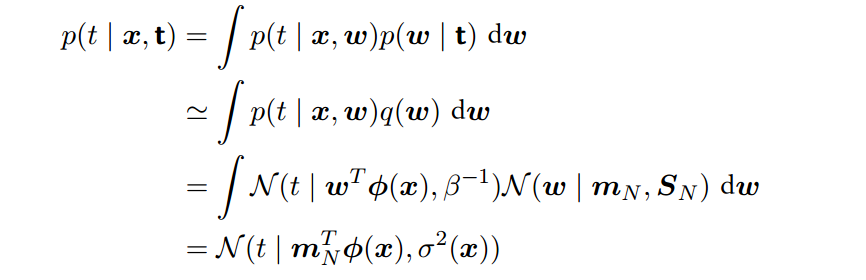# 变分推断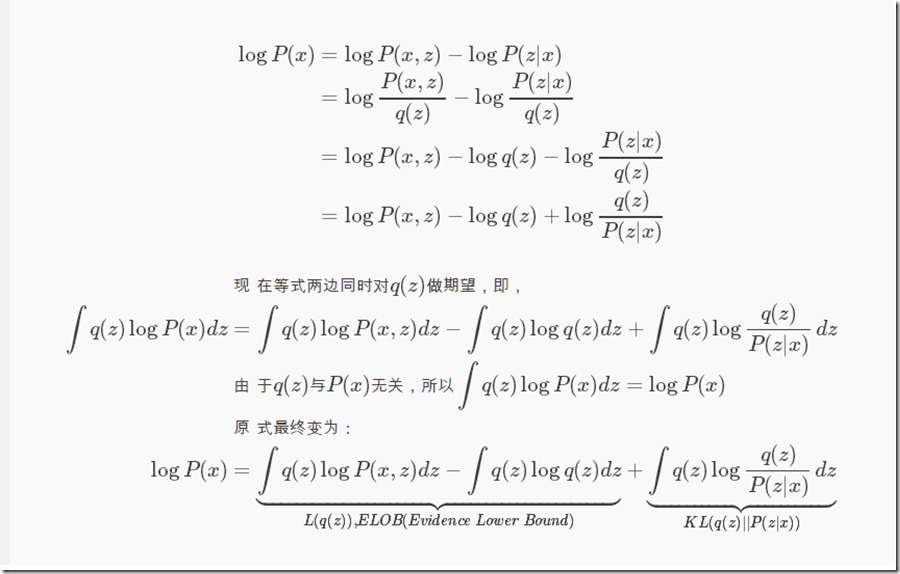KL divergence是衡量两个函数的不相似度，当等于0时表示两个分布完全一样。P(x)不变，那么想让KL( q || p )越小，即让ELOB越大，反之亦然。因为KL≥0，所以logP(x)≥ELOB。ELOB被称为变分下界。

## 平均场理论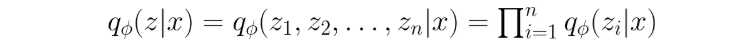## 变分下界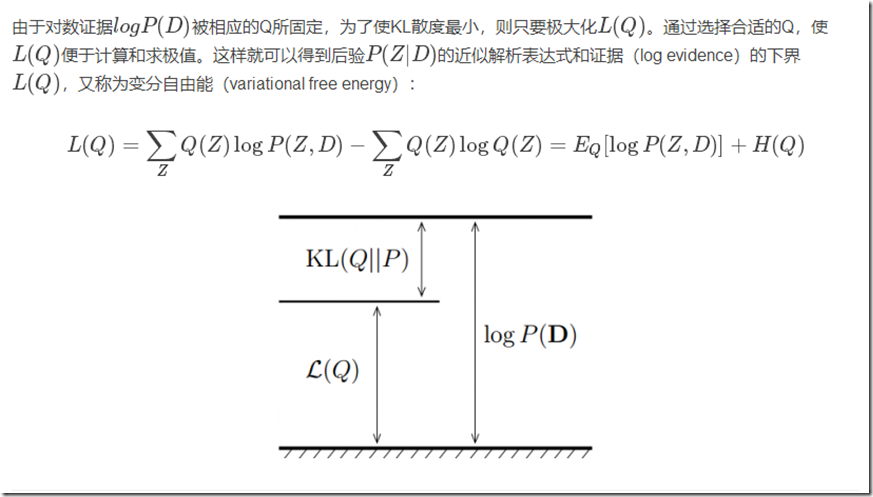## 求解过程（极大化ELOB）

$\mathcal L(q_j)=\int \prod_i q_j\{ lnp(X,Z)-\sum_ilnq_i \} dZ \\=\int q_j\{ \int ln(X,Z) \prod_{i\neq j}q_i dZ_i \} dZ_j -\int q_j ln q_jd Z_j +C \\ =\int q_j ln\check{p}(X,Z_j)dZ_j - \int q_j lnq_jdZ_j +C$

$=\int q_iln\check p(X,Z_j) dZ_j-\int q_jlnq_jdZ_j+C$

$q_j^*(Z_j)=\frac{\Bbb E_{i\neq j}[lnp(X,Z)]}{\int \Bbb E_{i\neq j}[lnp(X,Z)]dZ_j}$

# 一元高斯分布的例子

$p(D|μ,\tau) = (\frac{\tau}{ 2π })^{\frac{N}{2}}exp\{ -\frac{\tau}{2}\sum_{n=1}^{N} (x_n-μ)^2 \}$

$p(μ|\tau)=\mathcal{N}(μ|μ_0,(\lambda_0\tau)^-1) \\ \ \\ p(\tau)=Gam(\tau|a_0,b_0)$

$Gam(\lambda | a_0,b_0)=\frac{1}{\Gamma(a)} b^{a_0}_0\lambda^{a_0-1}exp(-b_0\lambda)$

$q(μ,\tau)=q_μ(μ)q_\tau(\tau)$

$lnq_μ^*(μ)=\Bbb E_{\tau}[lnp(D,μ|\tau)]+C \\=\Bbb E_{\tau}[lnp(D|μ,\tau)+ln(μ|\tau)]+C \\=\Bbb E_{\tau}[ -\frac{\tau}{2}\sum_{n=1}^{N} (x_n-μ)^2 +\frac{N}2 ln\frac{\tau}{2\pi}+\lambda_0(\mu-\mu_0)^2]+C \\=\Bbb E_{\tau}[ -\frac{\tau}{2}\sum_{n=1}^{N} (x_n-μ)^2 -\frac{\tau}{2}\lambda_0(\mu-\mu_0)^2]+C \\=-\frac12 \Bbb E[\tau]\{\lambda_0(\mu-\mu_0)^2+\sum_{n=1}^N(x_n-\mu)^2\}+C$现在将ln去掉，我们可以发现$q_\mu$为一个高斯分布$\mathcal{N}(\mu|\mu_N,\lambda_N^{-1})$均值和方差为：
$\mu_N=\frac{\lambda_0\mu_0+N\overline{x}}{\lambda_0+N} \\ \lambda_N=(\lambda_0+N)\Bbb E[\tau]$

$lnq_\tau^*(\tau)=\Bbb E_{\tau} [ lnp(D,μ|\tau) +lnp(\mu|\tau) ] +lnp(\tau)+C（7） \\其中lnp(\tau)=ln(\frac{1}{\Gamma(a)} b^{a_0}_0\lambda^{a_0-1}exp(-b_0\lambda)) \\=(a_0-1)ln\tau - b_0\tau+\frac{N+1}2ln\tau \\式子（7）=\Bbb E_{\tau}[ -\frac{\tau}{2}\sum_{n=1}^{N} (x_n-μ)^2 -\frac{\tau}{2}\lambda_0(\mu-\mu_0)^2]+C \\+(a_0-1)ln\tau - b_0\tau+\frac{N+1}2ln\tau$

# 例子：混合高斯的变分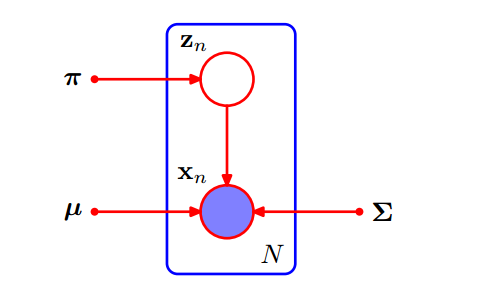$p(Z|π)=\prod_{n=1}^N\prod_{k=1}^K π_k^{z_nk}$

$p(X|Z,μ,\Lambda)=\prod_{n=1}^N\prod_{k=1}^K \mathcal{N}(x_n|μ_k,\Lambda_k^{-1})^{z_nk}$

$p(\pi)=Dir(\pi|\alpha_0)=C(\alpha_0)\prod_{k=1}^K \pi_k^{\alpha_0-1}$

$p(\mu,\Lambda)=p(\mu|\Lambda)p(\Lambda) \\=\prod_{k=1}^K\mathcal{N}(\mu_k|m_0,(\beta_0\Lambda_k)^{-1})\mathcal{W}(\Lambda_k|W_0,v_0)$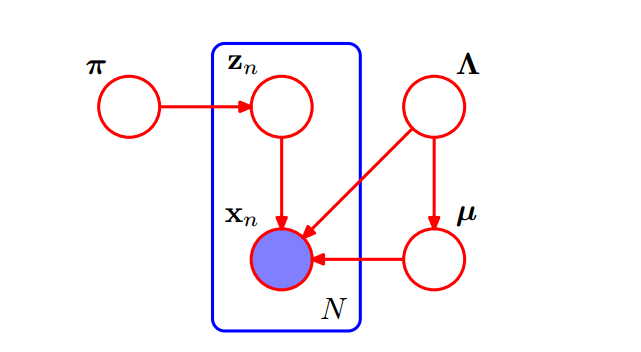## 变分分布

$p(X,Z,\pi,\mu,\Lambda)=p(X|Z,\mu,\Lambda)p(Z|\pi)p(\pi)p(\mu|\Lambda)p(\Lambda) （1）$

$q(Z,\pi,\mu,\Lambda)=q(Z)q(\pi,\mu,\Lambda) （2）$

$lnq^*(Z)=\Bbb E_{\pi,\mu,\Lambda}[lnp(X,Z,\pi,\mu,\Lambda)]+C（3）$

$lnq^*(Z)=\Bbb E_\pi[lnp(Z|\pi)]+\Bbb E_{\mu,\Lambda}[lnp(X|Z,\mu,\Lambda)]+C（4）$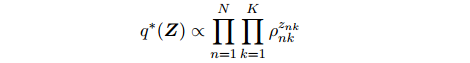$q^*(Z)=\prod_{n=1}^N\prod_{k=1}^K r_{nk}^{z_{nk}} \\其中: \\r_{nk}=\frac{\rho_{nk}}{\sum_{j=1}^k\rho_{nj}}$

$\Bbb E[z_{nk}]=r_{nk}$

$lnq^*(\pi,\mu,\Lambda)=lnp(\pi)+\sum_{k=1}^Klnp(\mu_k,\Lambda_k)+\Bbb E_Z[lnp(Z|\pi)] \\+\sum_{k=1}^K\sum_{n=1}^N\Bbb E[z_{nk}]ln\mathcal{N}(x_n|\mu_k,\Lambda_k^{-1})（6）$

$q(\pi,\mu,\Lambda)=q(\pi)\prod_{k=1}^K q(\mu_k,\Lambda_k) （7）$

$lnq^*(\pi)=(\alpha_0-1)\sum_{k=1}ln\pi_k+\sum_{k=1}^K\sum_{n=1}^Nr_{nk}ln\pi_k+C \\可以将q^*(\pi)看成是狄利克雷分布其中\alpha元素为\alpha_k，形式为：\\q^*(\pi)=Dir(\pi|\alpha_k) \\\alpha_k=\alpha_0+N_k$

$q^*(\mu_k,\Lambda_k)=\mathcal N(\mu_k|m_k,(\beta_k,\Lambda_k)^{-1})\mathcal W(\Lambda_k|W_k,v_k)$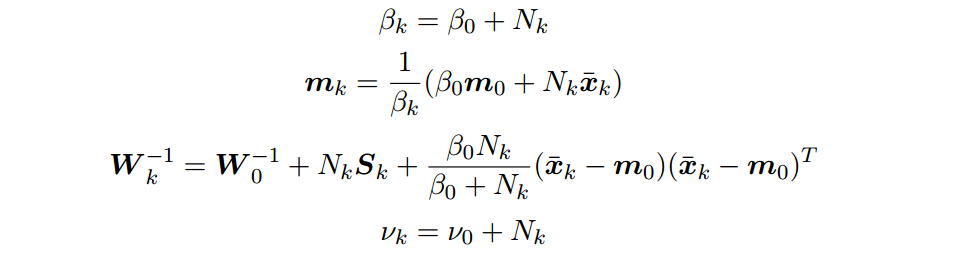## 变分下界

$\mathcal L(q_j)=\int q\{ lnp(X,Z)-\sum_ilnq_i \} dZ$

## 预测概率密度

$p(\check{x}|X)=\sum_{\check{z}}\iiint\pi_k\mathcal{N}(\check{x}|\mu_k,\Lambda_k^{-1})p(\pi,\mu,\Lambda|X)d\pi d\mu d \Lambda$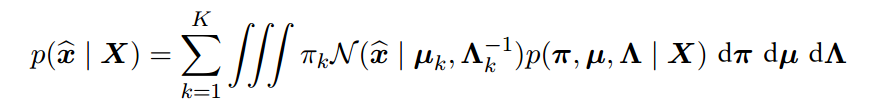$p(\check x|X)\backsimeq \sum_{k=1}^K\iiint\pi_k\mathcal N(\check x|\mu_k,\Lambda_k^{-1})q(\pi)q(\mu_k,\Lambda_k)d\pi d\mu_k d\Lambda_k$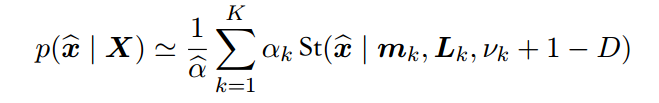# 变分线性回归

$\omega$的似然函数和先验分布为：
$p(t|\omega)=\prod_{n=1}^N\mathcal{N}(t_n|\omega^T\phi_n,\beta^{-1}) \\p(\omega|\alpha)=\mathcal{N}(\omega|0,\alpha^{-1}I) \\p(\alpha)=Gam(\alpha|a_0,b_0)$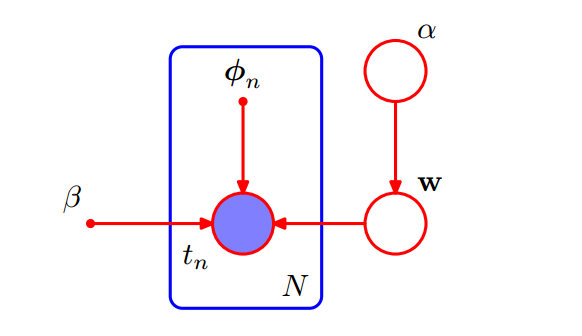$p(t,\omega,\alpha)=p(t|\omega)p(\omega|\alpha)p(\alpha)$

## 变分分布

$q(\omega,\alpha)=q(\omega)q(\alpha)$$lnq^*(\alpha)=lnp(\alpha)+\Bbb E_\omega[lnp(\omega|\alpha)]+C \\=(\alpha_0-1)ln\alpha-b_0\alpha+\frac M2ln\alpha-\frac{\alpha}2\Bbb E[\omega^T\omega]+C$

$q^*(\alpha)=Gam(\alpha|a_0+\frac M2,b_0+\frac 12\Bbb E[\omega^T\omega])$

$lnq^*(\omega)=lnp(t|\omega)+\Bbb E_\alpha[lnp(\omega|\alpha)]+c \\=-\frac\beta 2\sum_{n=1}^N\{ \omega^T\phi_n-t_n \}^2-\frac12\Bbb E[\alpha]\omega^T\omega+C \\=-\frac12\omega^T(\Bbb E[\alpha]I+\beta\Phi^T\Phi)\omega+\beta\omega^T\Phi^Tt+C$## 预测分布